## Overview: NLP Procedure

The NLP (nonlinear programming) procedure offers a set of optimization techniques for minimizing or maximizing a continuous nonlinear function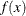ofdecision variables,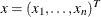with lower and upper bound, linear and nonlinear, equality and inequality constraints. This can be expressed as solving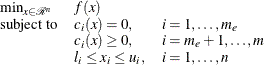where
is the objective function, the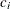’s are the nonlinear functions, and the’s and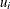’s are the lower and upper bounds. Problems of this type are found in many settings ranging from optimal control to maximum likelihood estimation.

The NLP procedure provides a number of algorithms for solving this problem that take advantage of a special structure on the objective function and constraints. One example is the quadratic programming problem,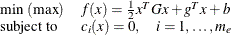where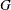is ansymmetric matrix,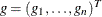is a vector,is a scalar, and the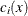’s are linear functions.

Another example is the least squares problem: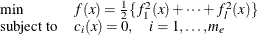where the’s are linear functions, and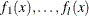are nonlinear functions of.

The following problems are handled by PROC NLP:

• quadratic programming with an option for sparse problems

• unconstrained minimization/maximization

• constrained minimization/maximization

• linear complementarity problem

The following optimization techniques are supported in PROC NLP:

• Trust Region Method

• Newton-Raphson Method with Line Search

• Newton-Raphson Method with Ridging

• Quasi-Newton Methods

• Double Dogleg Method

• Levenberg-Marquardt Method

• Hybrid Quasi-Newton Methods

These optimization techniques require a continuous objective function, and all but one (NMSIMP) require continuous first-order derivatives of the objective function. Some of the techniques also require continuous second-order derivatives. There are three ways to compute derivatives in PROC NLP:

• analytically (using a special derivative compiler), the default method

• via finite-difference approximations

• via user-supplied exact or approximate numerical functions

Nonlinear programs can be input into the procedure in various ways. The objective, constraint, and derivative functions are specified using the programming statements of PROC NLP. In addition, information in SAS data sets can be used to define the structure of objectives and constraints as well as specify constants used in objectives, constraints and derivatives.

PROC NLP uses data sets to input various pieces of information:

• The DATA= data set enables you to specify data shared by all functions involved in a least squares problem.

• The INQUAD= data set contains the arrays appearing in a quadratic programming problem.

• The INEST= data set specifies initial values for the decision variables, the values of constants that are referred to in the program statements, and simple boundary and general linear constraints.

• The MODEL= data set specifies a model (functions, constraints, derivatives) saved at a previous execution of the NLP procedure.

PROC NLP uses data sets to output various results:

• The OUTEST= data set saves the values of the decision variables, the derivatives, the solution, and the covariance matrix at the solution.

• The OUT= output data set contains variables generated in the program statements defining the objective function as well as selected variables of the DATA= input data set, if available.

• The OUTMODEL= data set saves the programming statements. It can be used to input a model in the MODEL= input data set.